# Calculate the difference between two dates in days, weeks, months and years in Excel

When working with dates in a worksheet, it may be necessary to determine the number of days, weeks, months, or years between two provided dates.

## Calculate the difference between two given dates in Days

You can use the following formulas to calculate the number of days that exist between two specific dates.

Step 1

At first, you must create a sample data as shown in following screenshot.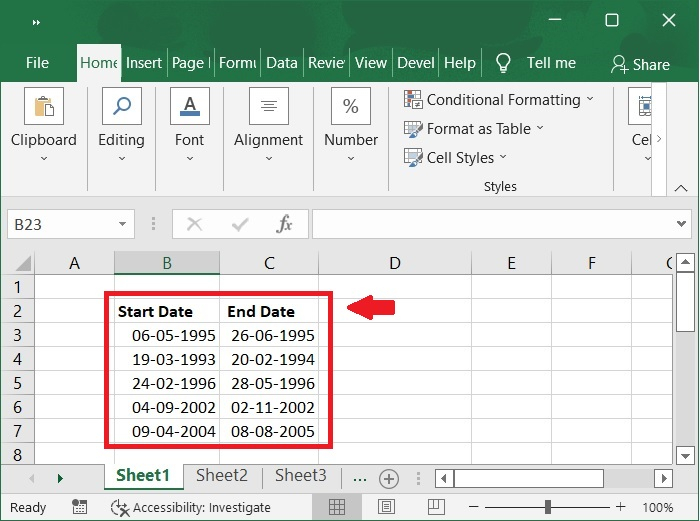Step 2

Any one of the following formulas should be entered into a blank cell to generate the correct result.

Formulas

=DATEDIF(B3,C3,"D")


Or,

=C3-B3


Here, B3 is Start Date and C3 End Date.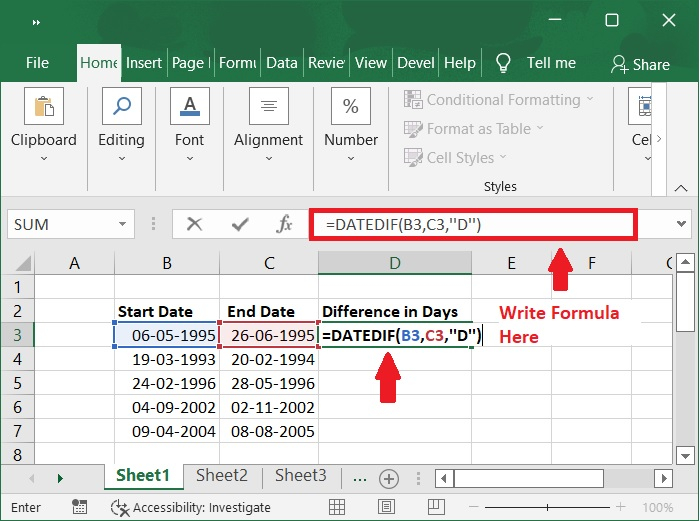Step 3

The number of days between the two dates will then be determined as seen in the screenshot below by dragging the fill handle downward into the cells.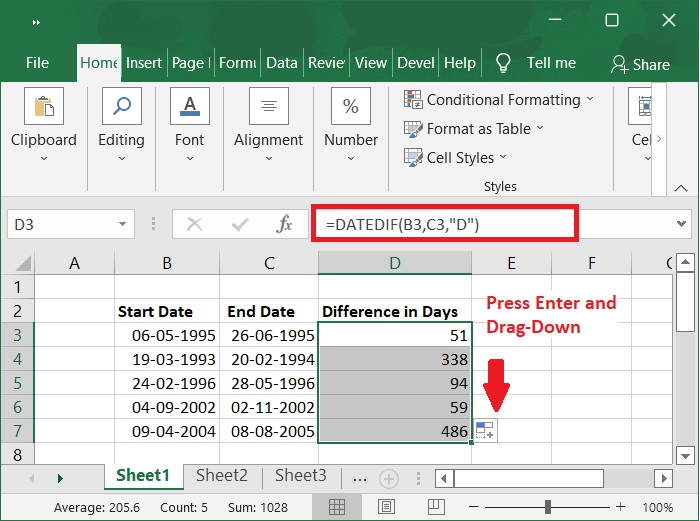## Calculate the difference between two given dates in Months

Let's see how to calculate the difference between two given dates in months.

Step 1

Enter the following formula into a blank field to determine how many months there are between two specific dates.

=DATEDIF(B3,C3,"M")


See the following screenshot.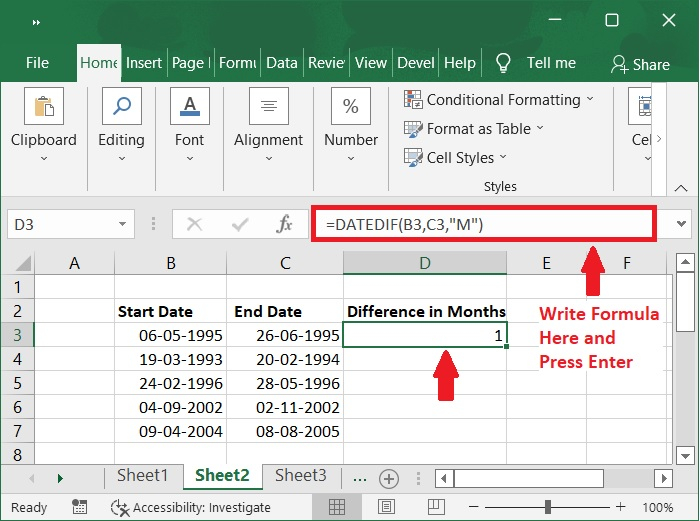Step 2

Then, drag the fill handle down to the cells to apply this formula, and the difference in months between two dates is determined as shown in the following screenshot.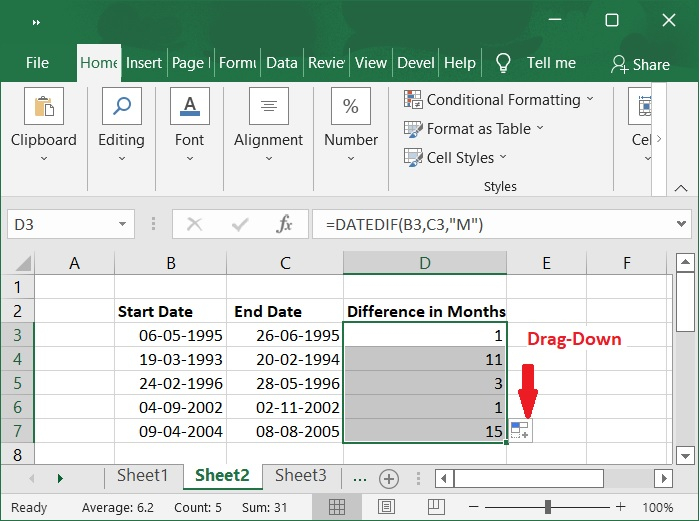## Calculate the difference between two given dates in Years

Now let's see how to calculate the difference between two given dates in years.

Step 1

The DATEDIF function can also be used to compute the number of years between two dates. Type the following formula into a cell that is empty.

=DATEDIF(B3,C3,"Y")


See the following screenshot.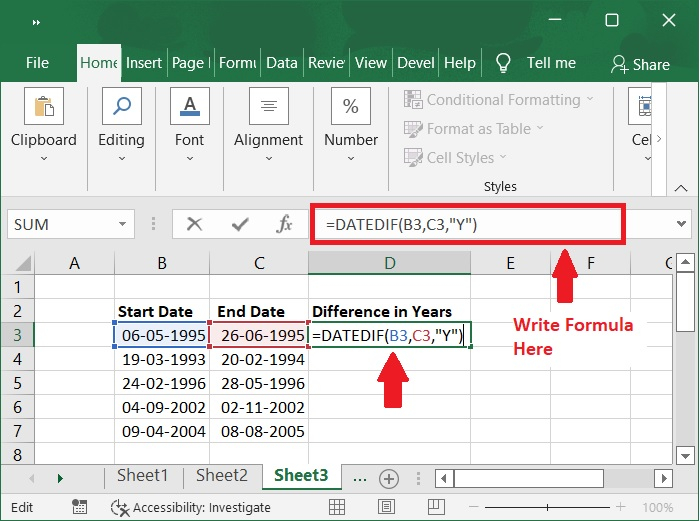Step 2

Then, drag the fill handle down to the cells to use this formula. The number of years between the two specific dates has been returned, as seen in the screenshot below.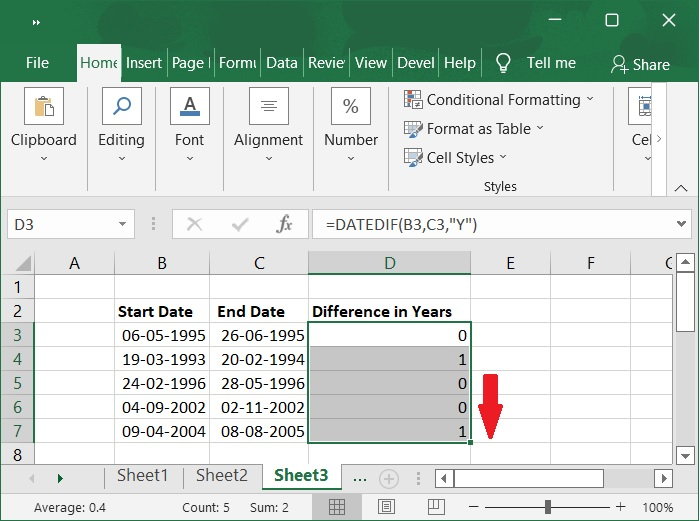## Calculate the difference between two given dates in Days, Months and Year

Follow the steps given below to calculate the difference between two given dates in days, months, and year.

Step 1

If you need to figure out the difference in days, months, and years between two dates, use the formula given below.

=DATEDIF(B3, C3, "y") &" years, "&DATEDIF(B3, C3, "ym") &"
months, " &DATEDIF(B3, C3, "md") &" days"


See the following screenshot.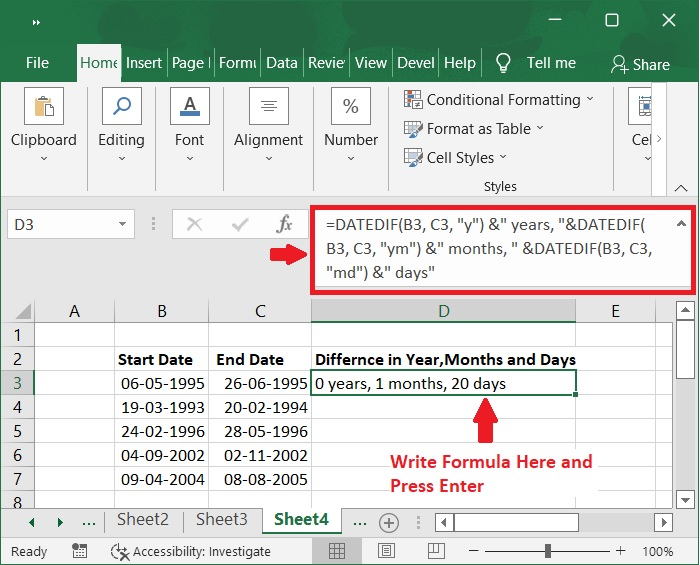Step 2

The fill handle to the cells where you wish to apply this formula, and then drag it down.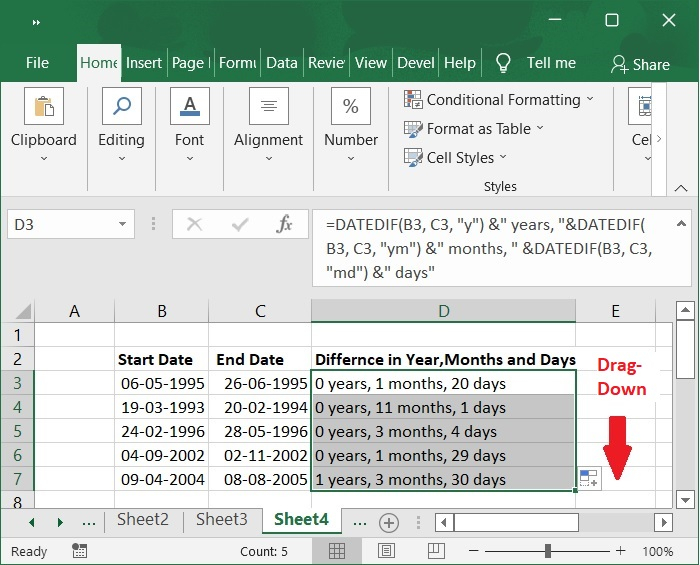## Calculate the difference between two given dates in Weeks

Follow the steps given below to calculate the difference between two given dates in weeks.

Step 1

Use the following formula to determine the number of full weeks between two dates.

=ROUNDDOWN((DATEDIF(B3, C3, "d") / 7), 0)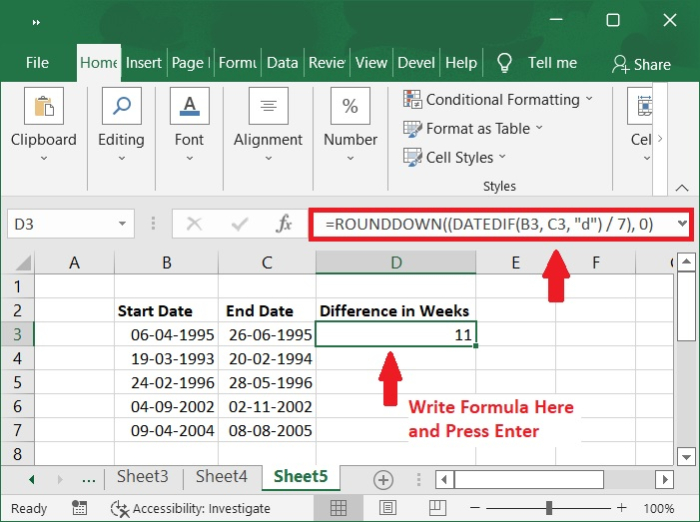Step 2

Next, drag the fill handle to the cells where you wish to apply this formula by dragging it downward.## Conclusion

In this tutorial, we explained in detail how you can calculate the difference between two dates in days, months, years, weeks in Excel.